Stack and Crop Raster Data Using EarthPy

Learn how to stack and crop satellite imagery using EarthPy

Stack and Crop Raster Data Using EarthPy

Note

The examples below will show you how to use the es.stack() and es.crop_image() functions from EarthPy.

Stack Multi Band Imagery

Some remote sensing datasets are stored with each band in a separate file. However, often you want to use all of the bands together in your analysis. For example you need all of the bands together in the same file or “stack” in order to plot a color RGB image. EarthPy has a stack() function that allows you to take a set of .tif files that are all in the same spatial extent, CRS and resolution and either export them together a single stacked .tif file or work with them in Python directly as a stacked numpy array.

To begin using the EarthPy stack() function, import the needed packages and create an array to be plotted. Below you plot the data as continuous with a colorbar using the plot_bands() function.

Import Packages

You will need several packages to stack your raster. You will use GeoPandas to open up a shapefile that will be used to crop your data. You will primarily be using the EarthPy spatial module in this vignette.

import os
from glob import glob
import matplotlib.pyplot as plt
import rasterio as rio
from rasterio.plot import plotting_extent
import geopandas as gpd
import earthpy as et
import earthpy.spatial as es
import earthpy.plot as ep

Get Example Data Ready for Stack

With EarthPy you can create a stack from all of the Landsat .tif files (one per band) in a folder with the es.stack() function.

Error found on Windows systems

Note

If you are running this script on a Windows system, there is a known bug with .to_crs(), which is used in this script. If an error occurs, you have to reset your os environment with the command os.environ["PROJ_LIB"] = r"path-to-share-folder-in-environment".

# Get sample data from EarthPy and setting your home working directory

data_path = et.data.get_data("vignette-landsat")
os.chdir(os.path.join(et.io.HOME, "earth-analytics"))

# Prepare the landsat bands to be stacked using glob and sort

landsat_bands_data_path = "data/vignette-landsat/LC08_L1TP_034032_20160621_20170221_01_T1_sr_band*[2-4]*_crop.tif"
stack_band_paths = glob(landsat_bands_data_path)
stack_band_paths.sort()

# Create output directory and the output path

output_dir = os.path.join("data", "outputs")
if os.path.isdir(output_dir) == False:
os.mkdir(output_dir)

raster_out_path = os.path.join(output_dir, "raster.tiff")

Stack the Bands

The stack function has an optional output argument, where you can write the raster to a tiff file in a folder. If you want to use this functionality, make sure there is a folder to write your tiff file to. The Stack function also returns two object, an array and a RasterIO profile. Make sure to be catch both in variables.

# Stack Landsat bands

os.chdir(os.path.join(et.io.HOME, "earth-analytics"))
array, raster_prof = es.stack(stack_band_paths, out_path=raster_out_path)

Create Extent Object

To get the raster extent, use the plotting_extent function on the array from es.stack() and the Rasterio profile or metadata object. The function needs a single layer of a numpy array, which is why we use arr. The function also needs the spatial transformation for the Rasterio object, which can be acquired by accessing the "transform" key within the Rasterio Profile.

extent = plotting_extent(array, raster_prof["transform"])

Plot Un-cropped Data

You can see the boundary and the raster before the crop using ep.plot_rgb() Notice that the data appear washed out.

fig, ax = plt.subplots(figsize=(12, 12))
ep.plot_rgb(
array,
ax=ax,
stretch=True,
extent=extent,
str_clip=0.5,
title="RGB Image of Un-cropped Raster",
)
plt.show()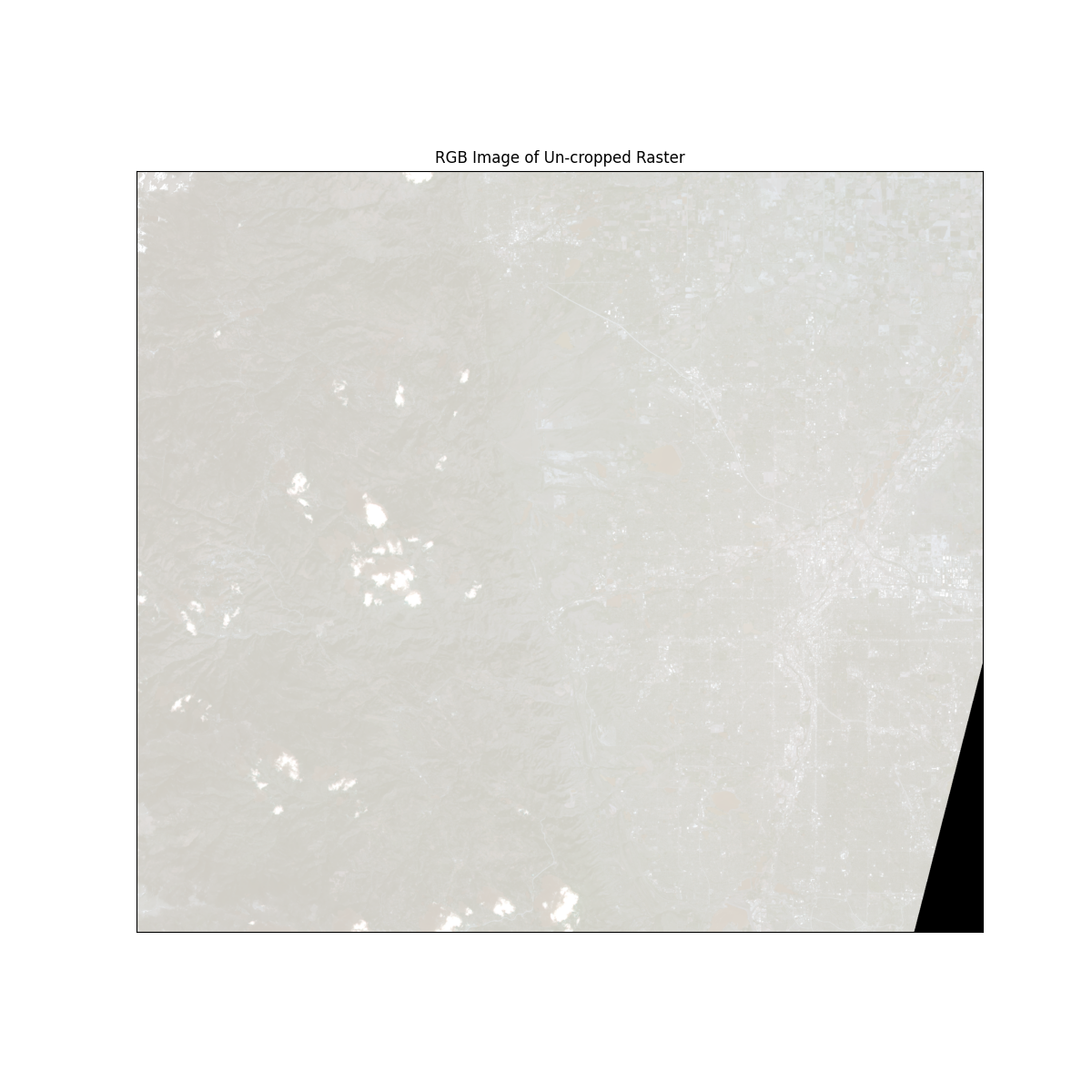Explore the Range of Values in the Data

You can explore the range of values found in the data using the EarthPy hist() function. Do you notice any extreme values that may be impacting the stretch of the image?

ep.hist(array, title=["Band 1", "Band 2", "Band 3"])
plt.show()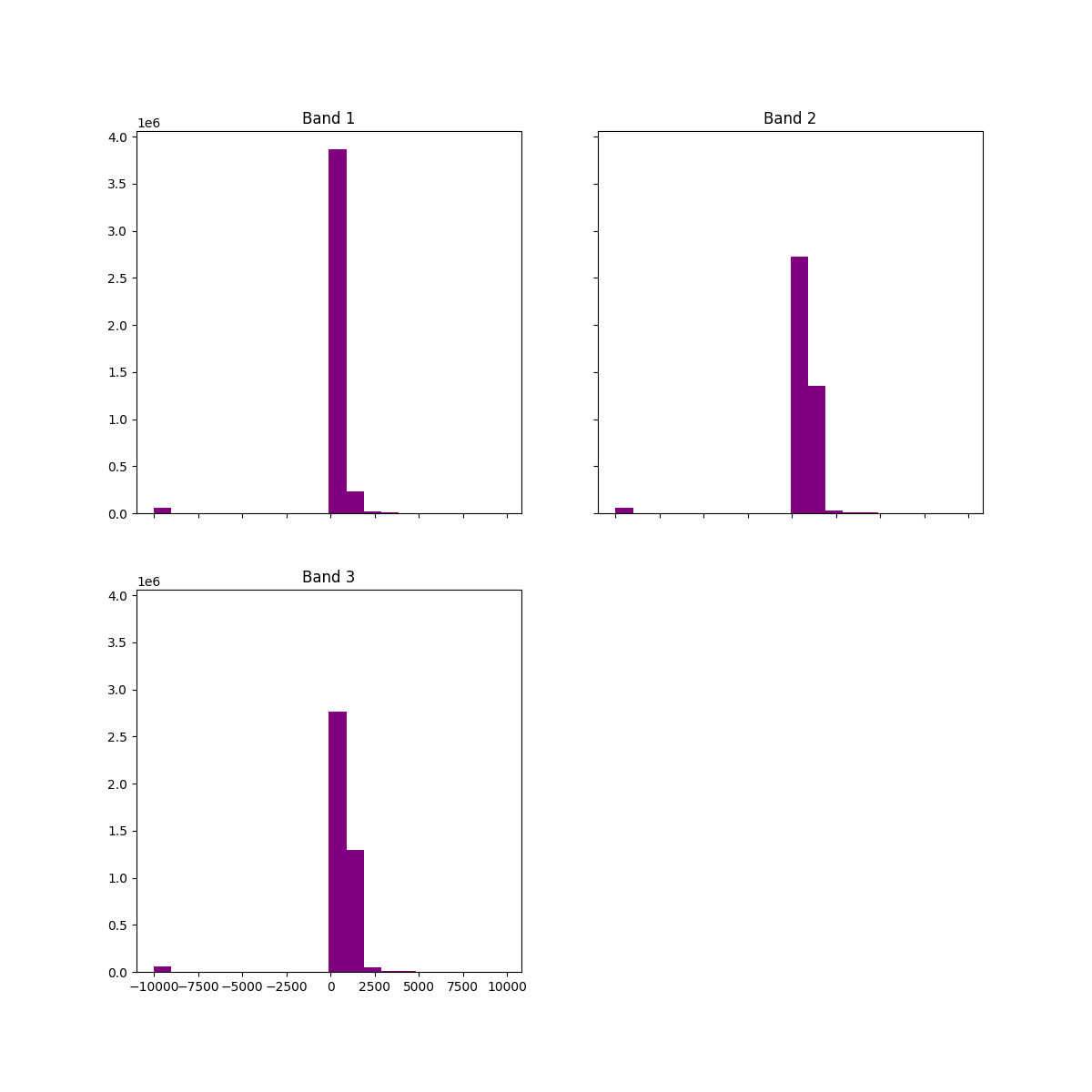No Data Option

es.stack() can handle nodata values in a raster. To use this parameter, specify nodata=. This will mask every pixel that contains the specified nodata value. The output will be a numpy masked array.

os.chdir(os.path.join(et.io.HOME, "earth-analytics"))
array_nodata, raster_prof_nodata = es.stack(stack_band_paths, nodata=-9999)

# View hist of data with nodata values removed
ep.hist(
array_nodata,
title=[
"Band 1 - No Data Values Removed",
"Band 2 - No Data Values Removed",
"Band 3 - No Data Values Removed",
],
)
plt.show()

# Recreate extent object for the No Data array

extent_nodata = plotting_extent(
array_nodata, raster_prof_nodata["transform"]
)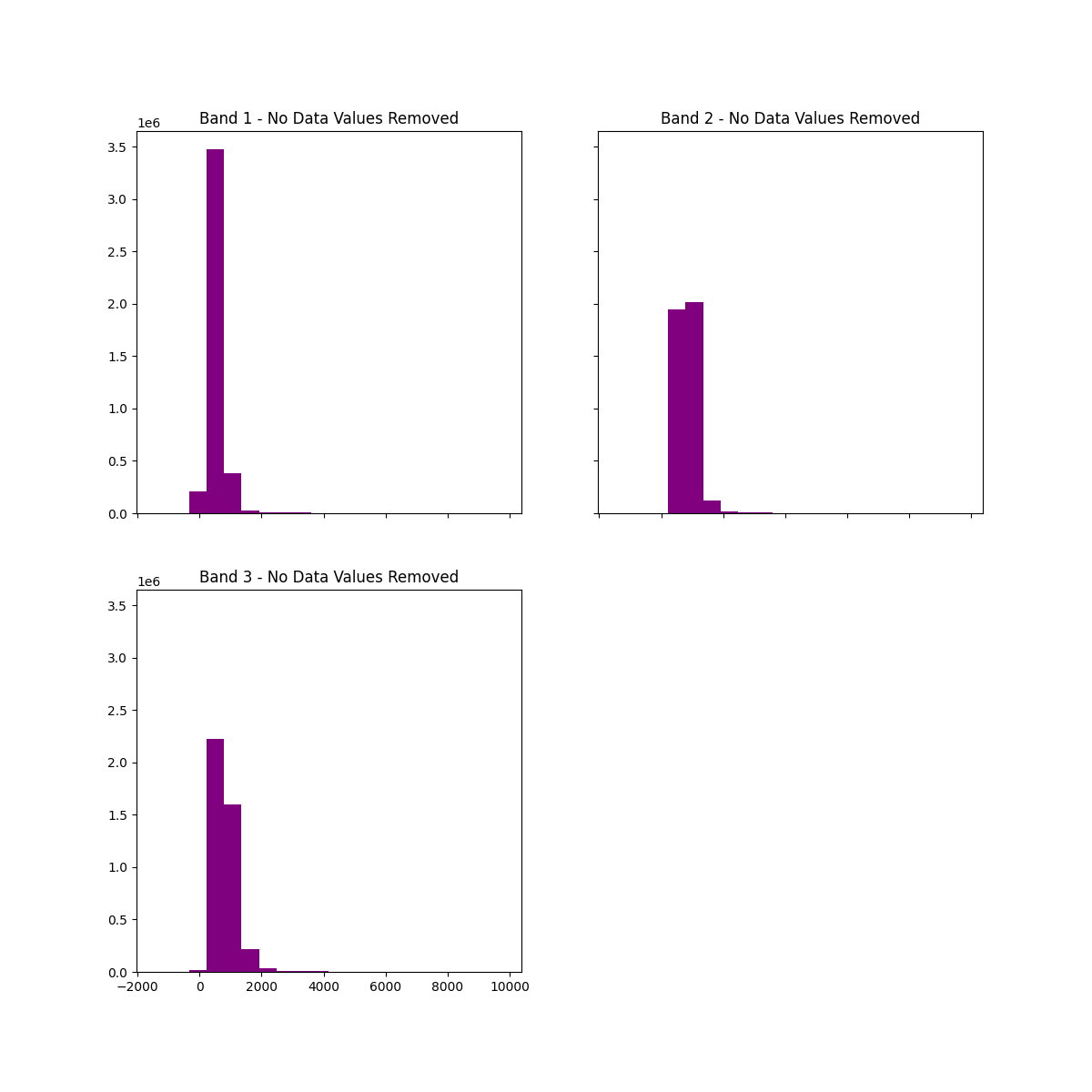Plot Un-cropped Data

Plot the data again after the nodata values are removed.

fig, ax = plt.subplots(figsize=(12, 12))
ep.plot_rgb(
array_nodata,
ax=ax,
stretch=True,
extent=extent,
str_clip=0.5,
title="RGB image of Un-cropped Raster, No Data Value Selected",
)
plt.show()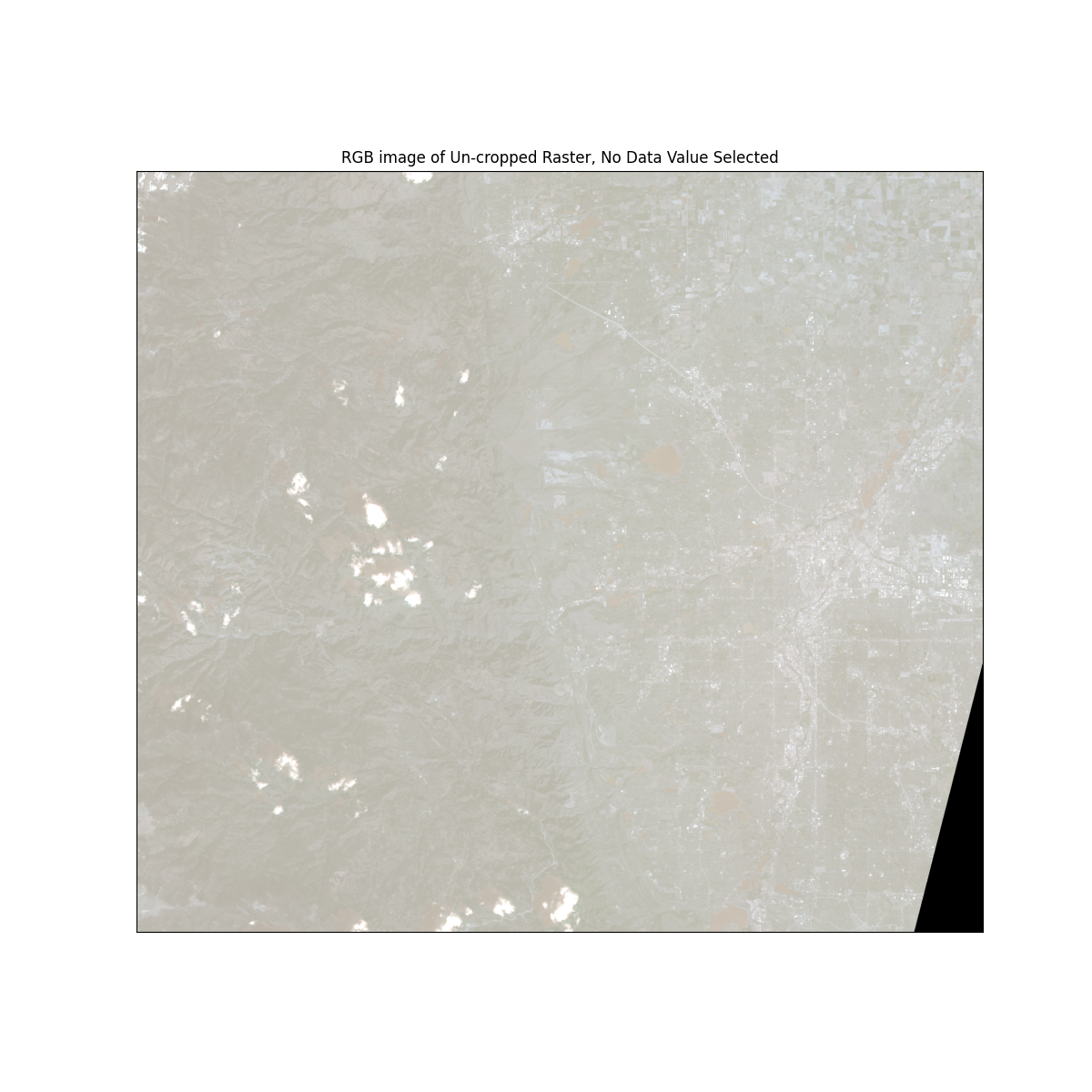Crop the Data

Sometimes you have data for an area that is larger than your study area. It is more efficient to first crop the data to your study area before processing it in Python. The fastest and most efficient option is to crop each file individually, write out the cropped raster to a new file, and then stack the new files together. To do this, make sure you have a ShapeFile boundary in the form of a GeoPandas object you can use as the cropping object. Then, loop through every file you wish to crop and crop the image, then write it out to a file. Take the rasters created and stack them like you stacked bands in the previous examples.

os.chdir(os.path.join(et.io.HOME, "earth-analytics"))

# Open the crop boundary using GeoPandas.

"data/vignette-landsat/vector_layers/fire-boundary-geomac/co_cold_springs_20160711_2200_dd83.shp"
)

Reproject the data

Note

If you are on windows, make sure to set your environment here!

The crop function won’t work properly if the data are in different Coordinate Reference Systems (CRS). To fix this, be sure to reproject the crop layer to match the CRS of your raster data. To reproject your data, first get the CRS of the raster from the rasterio profile object. Then use that to reproject using geopandas .to_crs method.

os.chdir(os.path.join(et.io.HOME, "earth-analytics"))

with rio.open(stack_band_paths) as raster_crs:
crop_raster_profile = raster_crs.profile
crop_bound_utm13N = crop_bound.to_crs(crop_raster_profile["crs"])

Crop Each Band

When you need to crop and stack a set of images, it is most efficient to first crop each image, and then stack it. es.crop_all() is an efficient way to crop all bands in an image quickly. The function will write out cropped rasters to a directory and return a list of file paths that can then be used with es.stack().

os.chdir(os.path.join(et.io.HOME, "earth-analytics"))

band_paths_list = es.crop_all(
stack_band_paths, output_dir, crop_bound_utm13N, overwrite=True
)

Stack All Bands

Once the data are cropped, you are ready to create a new stack.

os.chdir(os.path.join(et.io.HOME, "earth-analytics"))

cropped_array, array_raster_profile = es.stack(band_paths_list, nodata=-9999)
crop_extent = plotting_extent(
cropped_array, array_raster_profile["transform"]
)

# Plotting the cropped image
# sphinx_gallery_thumbnail_number = 5
fig, ax = plt.subplots(figsize=(12, 6))
crop_bound_utm13N.boundary.plot(ax=ax, color="red", zorder=10)
ep.plot_rgb(
cropped_array,
ax=ax,
stretch=True,
extent=crop_extent,
title="Cropped Raster and Fire Boundary",
)
plt.show()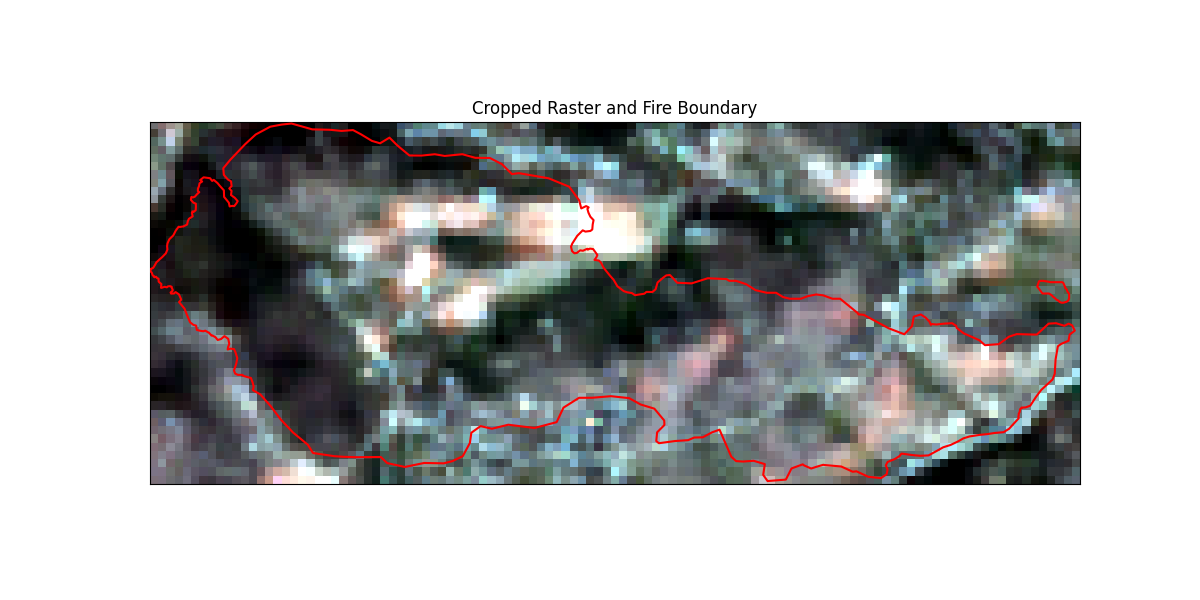Crop Individual Bands

If you only need to crop one raster image, you can use EarthPy’s es.crop_image() function. This function takes a Rasterio object and crops it to the provided spatial extent.

# Open Landsat image as a Rasterio object in order to crop it
os.chdir(os.path.join(et.io.HOME, "earth-analytics"))

with rio.open(stack_band_paths) as src:
single_cropped_image, single_cropped_meta = es.crop_image(
src, crop_bound_utm13N
)

# Create the extent object
single_crop_extent = plotting_extent(
single_cropped_image, single_cropped_meta["transform"]
)

# Plot the newly cropped image
fig, ax = plt.subplots(figsize=(12, 6))
crop_bound_utm13N.boundary.plot(ax=ax, color="red", zorder=10)
ep.plot_bands(
single_cropped_image,
ax=ax,
extent=single_crop_extent,
title="Single Cropped Raster and Fire Boundary",
)
plt.show()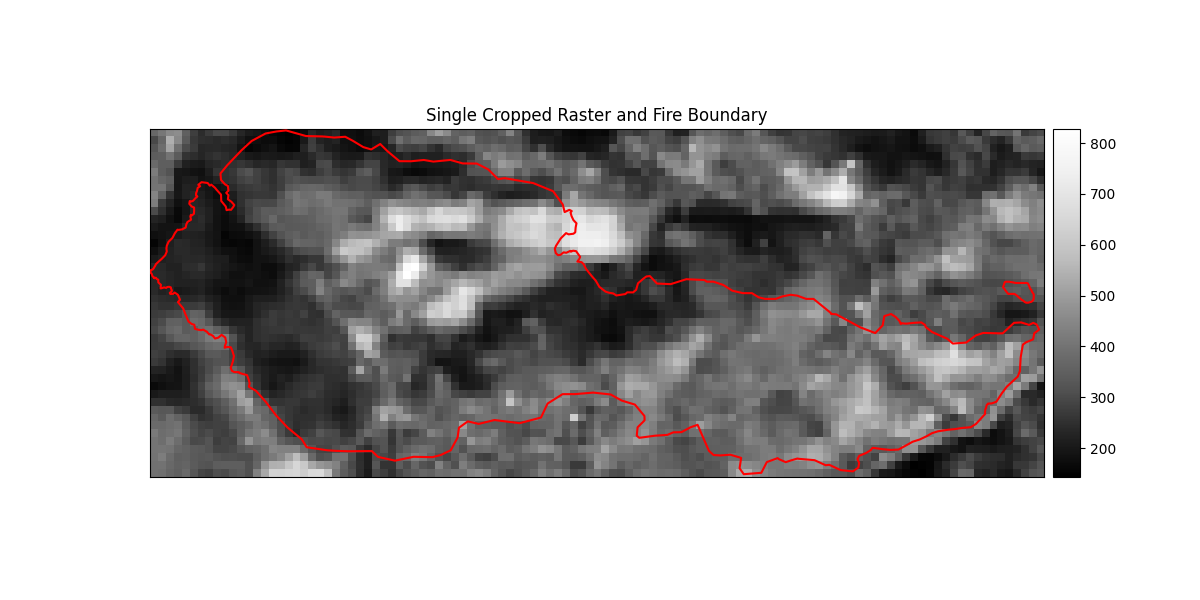Total running time of the script: ( 0 minutes 5.463 seconds)

Gallery generated by Sphinx-Gallery